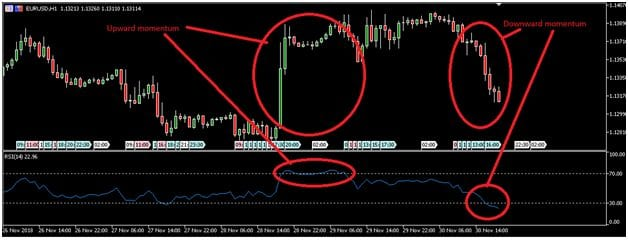• 0

# How does Relative Strength Index (RSI) work?

• 0

## Recommended Posts

• 0
Relative Strength Index (RSI) is an oscillator that measures price momentum. It can be used for multiple purposes in Forex, including to identify overbought or oversold conditions, find impending trend reversals, and measure the strength of a trend.

## Relative Strength Index explained

There are three components that are used to calculate RSI: Average Gain, Average Loss, and Relative Strength (RS). The first point of Average Gain is calculated by adding together the last 14 periods of gains and dividing them by 14. For each subsequent period, the Average Gain is calculated by taking the previous period’s average gain and multiplying it by 13, then adding the current gain and dividing the total by 14. Average Loss is calculated the same way. The first point is simply the average of all losses over the past 14 periods. Each subsequent point adds this period’s loss to the previous one and then divides the total by 14. Relative Strength (RS) is calculated by taking the Average Gain and dividing it by the Average Loss. When this number is put through a smoothing function, it results in a percentage value between 0 and 100. This percentage is called RSI. RSI is a measurement of how quickly price is moving. If RSI is above 70, price is moving upward at a rapid rate. If RSI is below 30, price is falling quickly. If RSI is 50, price is either range bound or trending slowly.

## RSI exampleIn this hourly chart of EUR/USD, the price rose rapidly between 3 p.m. Nov. 28 and 7 a.m. Nov. 29. At the same time, RSI rose from 40 to above 70. The price then began to consolidate. As a result, RSI moved below 70. On the morning of November 30, the price then began to fall, and it fell faster as the day continued. As a result, RSI moved below 30.

## How to trade using RSI

There are multiple ways that traders can use RSI, including to identify overbought/oversold conditions, confirm a strong trend, find divergences, and find failure swings.

## Using RSI to identify overbought and oversold conditions

If RSI is above 70, the currency pair is considered “overbought.” This means the price is moving up too rapidly and the rally is likely to consolidate soon. A trader can use this signal to exit a long trade or to start looking for opportunities to short. If RSI is below 30, it is considered “oversold.” In this case, traders may want to consider exiting short trades and getting ready for opportunities to go long.

## Using RSI to confirm a trend

If another indicator suggests that a breakout is occurring, RSI can be used to confirm that the trend is strong enough be useful. In this case, RSI should be above 50% in an uptrend or below 50% in a downtrend. If the price is rising but RSI is not above 50% or if the price is falling but RSI is not below 50%, there is a greater chance that the rally or retreat will be short-lived.

## Using RSI to find divergences

If RSI is moving up while price is moving down, this is a bullish divergence and indicates a possible reversal upward. If the RSI is moving down while price is moving up, this is a bearish divergence and indicates a possible reversal downward.

## Using RSI to find failure swings

If RSI moves below 30, rises above 30, then pulls back and holds without going below 30 again, a subsequent move above its previous high is a “bullish failure swing.” That is a signal to buy. Likewise if RSI moves above 70, falls below 70, then pulls up again without moving above 70, a subsequent break below its previous low is a “bearish failure swing.” That is a signal to sell. RSI measures a ratio of the average gain and average loss over a period of time. It can be used to find entry and exit points and recognize impending reversals. That is how relative Strength Index works.

## Join the conversation

You can post now and register later. To reply to this question, sign in or create a new account.×   Pasted as rich text.   Paste as plain text instead

Only 75 emoji are allowed.

×   Your previous content has been restored.   Clear editor

×   You cannot paste images directly. Upload or insert images from URL.

×